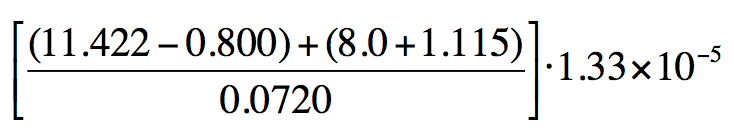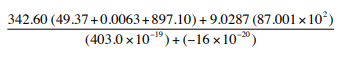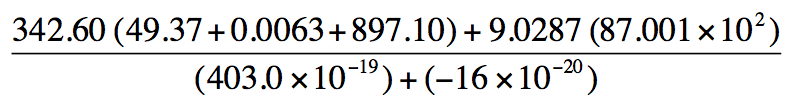Start typing, then use the up and down arrows to select an option from the list.# Analytical Chemistry

Learn the toughest concepts covered in your Analytical Chemistry class with step-by-step video tutorials and practice problems.

Experimental Error

# Multiplication and Division Operations

Multiplication and division of values written in scientific notation.

## Multiplication and Division

When multiplying or dividing your final answer will have the least number of significant figures.

1

#### concept

Multiplication and Division Operations1m
Play a video:
So when you multiply values in scientific notation, remember that you multiply the coefficients and you're gonna add the exponents. So here we have eight times 10 to the X times b times 10 to the Y. So you multiply the coefficients together and then we add the exponents together. So it'd be X plus Y. Now when we're dividing we're gonna divide the coefficients and you're going to subtract the exponents. So here this would become a divided by B Times 10 X -Y. Now, after multiplying and or dividing, remember that the coefficients will have the least significant figures doing that will give us our our most basic answer, our most correct answer at the end of our calculations. So applying what we've just learned, try to solve example one come back and see how I approached that same problem. Remember use the methods that are described up above to try to answer this question correctly.
2

#### example

Multiplication and Division1m
Play a video:
So here we're gonna say using the methods discussed above, we're gonna attempt to answer this question here. So remember when we're multiplying or dividing our coefficients need to have the least number of sig figs. So our coefficients are here. So when we multiply those three coefficients together we get initially 10.4, times 10 with our exponents here, we're gonna have to add them together. Five plus minus three plus six here. So five plus minus three plus six here gives us eight. So it's gonna be times 10 to the eight. Remember when it comes to your coefficient? It has to be a value that is between that is between one and 10, it has to be a value that's equal to or greater than one but less than 10. So I'm gonna move this this decimal point over one to make it one point something. by decreasing it by one, I have to increase my exponent by one. So now this becomes 1.4822 times 10 to the nine. Now our coefficients, this one here has four sig figs. This one here has two sig figs. And this one here has three sig figs. We want the least number of significant figures for coefficient. So I have to So it's gonna come 1.0 times 10 to the nine as our final answer here. So just remember to follow the steps and techniques that we've used thus far in order to get the correct answer. Not that you've seen that one attempt to do example to once you've done that come back and see how I approach that same exact question.
3

#### example

Multiplication and Division1m
Play a video:
in this one. We have mixed operations but the methods are still the same. We're gonna first figure out. Well my answer will be when I multiply these two coefficients, then divide by this one here. So when we do those methods we get 11.8454. And then remember when you're multiplying you add the exponents together and when you're dividing you subtract them. So multiplying times 10 to the eight times 10 to negative one. We're really adding those together. That's 10 to the seventh, divided by 10 to the 11th, Which is really 7 -11 which gives us -4. So that's gonna be times 10 to the -4. Remember we need to write our scientific notation where the coefficient is between one and 10. So I'm gonna move this decimal over one because it decreased by one. That means my exponent has to increase by one. So it becomes 1.18454 times 10 to the minus three. In terms of our coefficients here, this one has three significant figures. This one here has three significant figures and this one here also has three significant figures. So my answer at the end would be 1.18 times 10 to the -3. That's my final answer. So just remember when we're multiplying versus when we're dividing, what are the proper operations that we need to do in order to get our final answer

## Multiplication and Division Calculations

4

#### example

Multiplication and Division Calculations3m
Play a video:
5
Problem

Perform the following calculation for the image below to the right number of sig figs:

[(11.422-0.800) + (8.0 + 1.115)/0.0720] 1.33 x 10-56
Problem

Compute the following and determine the correct number of significant figures in the answer for the image below: